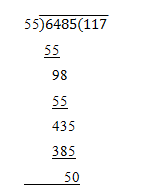# Number Systems ( 16 to 20 )

#### Prev     Next

Number Systems ( 16 to 20 ):

# APTITUDE QUESTIONS (NUMBER SYSTEMS):

16. How many natural numbers are there between 22 and 100 which are exactly divisible by 5?

A)    6

B)    12

C)    15

D)    13

E)    None of these

Explanation:

The required numbers are 25, 30, 35, 40,…., 95.

This is an A.P. in which a = 25, d = 5 and l = 95

Let the number of terms in it be n

Then, tn = 95

• a + (n – 1)d = 95
• 25 + (n – 1) * 5 = 95
• (n – 1) * 5 = 70
• (n – 1) = 14
• n = 15

17. If the number 5 * 2 is divisible by 6, then * = ?

A)    9

B)    8

C)    2

D)    7

E)    None of these

Explanation:

6 = 3 * 2.

So, 5 * 2 is divisible by 2.

Replace * by x

Then, (5 + x + 2) must be divisible by 3.

So, x = 2.

18. The sum of even numbers between 1 and 31 is:

A)    6

B)    128

C)    240

D)    512

E)    None of these

Explanation:

Let Sn = (2 + 4 + 6 + ….. + 30). This is an A.P. in which a = 2, d = 2, and l = 30

Let the number of terms be n. Then,

• a + (n – 1)d = 30
• 2 + (n – 1) * 2 = 30
• n = 15

∴ Sn = n/2 (a + l) = 15/2 * (2 + 30) = 15 * 16 = 240

19. Which one of the following cannot be the square of a natural number?

A)    32761

B)    81225

C)    32437

D)    20164

E)    None of these

Explanation:

The square of a natural number never ends in 2, 3, 7 or 8

∴ 32437 is not the square of a natural number.

20. Which natural number is nearest to 6485, which is completely divisible by 55?

A)    6435

B)    6500

C)    6550

D)    6525

E)    None of these

Explanation:∴ required number = 6485 – 50 = 6435